####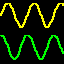2. Sinusoids4

In this lab we introduce the fundamentals of Matlab. Matlab is a programming environment that you will find helpful for many of the exercises in this text.
Manipulating sinusoid functions using complex exponentials turns trigonometric problems into simple arithmetic and algebra. In this lab, we first review the complex exponential signal and the phasor addition property needed for adding cosine waves. Then we will use Matlab to make plots of phasor diagrams that show the vector addition needed when combining sinusoids.
Manipulating sinusoid functions using complex exponentials turns trigonometric problems into simple arithmetic and algebra. In this lab, we first review the complex exponential signal and the phasor addition property needed for adding cosine waves. Then we will use Matlab to make plots of phasor diagrams that show the vector addition needed when combining sinusoids. [Files]
The objective of this lab is to learn how the outputs from two spatially separated sensors that receive signals from the same source can be used to estimate the direction to the source of the signal. The key to this processing is phase difference or time difference of arrival (TDOA) at the two receivers. [Files]

####3. Spectrum Representation7

In this lab, we will synthesize more complicated sinusoidal waveforms composed of sums of sinusoidal signals, each with a different frequency. The sounds synthesized will one of several songs. [Files]
In this lab, we will synthesize more complicated sinusoidal waveforms composed of sums of sinusoidal signals, each with a different frequency. The sounds synthesized will sound like a voice. [Files]
The objective of this lab is to introduce more complicated signals that are related to the basic sinusoid. These are signals which implement frequency modulation (FM) and amplitude modulation (AM) are widely used in communication systems such as radio and television, but they also can be used to create interesting sounds that mimic musical instruments.
This lab includes a project on sound synthesis with sinusoids. The sound synthesis will be done with sinusoidal waveforms of the form $$x(t) = \sum_k A_k \cos(\omega_k t + \phi_k)$$ where the amplitudes can be manipulated to produce a musical illusion. The challenge of the lab is to adjust the amplitudes to improve the subjective quality for listening.
The objective of this lab is to introduce more complicated signals that are related to the basic sinusoid. These are signals which implement frequency modulation (FM) and amplitude modulation (AM) are widely used in communication systems such as radio and television, but they also can be used to create interesting sounds that mimic musical instruments. [Files]
The objective of this lab is to introduce more complicated signals that are related to the basic sinusoid. These signals which implement frequency modulation (FM) and amplitude modulation (AM) are widely used in communication systems such as radio and television. In addition, they can be used to create interesting sounds that mimic musical instruments. The resulting signal can be analyzed to show its time-frequency behavior by using the spectrogram. This lab studies signal synthesis for AM and FM signals, and their time-frequency content as shown in a spectrogram. An underlying objective of the lab is to learn more about the spectrogram.
In this lab, we will synthesize more complicated sinusoidal waveforms composed of sums of sinusoidal signals, each with a different frequency. The sounds synthesized will one of several songs.

####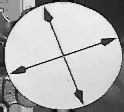4. Sampling and Aliasing2

The objective in this lab is to introduce digital images as a second useful signal type. We will show how the A-to-D sampling and the D-to-A reconstruction processes are carried out for digital images. In particular, we will show a commonly used method of image zooming (reconstruction) that gives poor results a later lab will revisit this issue and do a better job. [Files]
The objective of this lab is to study further the spectral content of signals analyzed via the spectrogram. There are several specific steps that will be considered in this lab:
1. Synthesize a linear-FM chirp with a Matlab M-file, and display its spectrogram. Choose the chirp parameters so that aliasing will happen.
2. Synthesize a periodic triangle wave with a Matlab M-file, and display its spectrogram. Relate the harmonic line spectrum to the fundamental period of the triangle wave.
3. Compare spectrograms using different scales for amplitude: decibels (dB) for amplitude versus linear amplitude.
4. Examine details of the harmonic lines in the dB spectrogram of the triangle wave.
5. Spectrogram: make a spectrogram of your voice signal, and relate the harmonic line spectrum to your previous measurement of pitch period.

####5. FIR Filters4

The goal of this lab is to learn how to implement FIR filters in Matlab, and then study the response of FIR filters to various signals, including images and speech. As a result, you should learn how filters can create interesting effects such as blurring and echoes. In addition, we will use FIR filters to study the convolution operation and properties such as linearity and time-invariance. [Files]
The goal of this lab is to learn how to implement FIR filters in Matlab, and then study the response of FIR filters to various signals, including images or speech. [Files]
The goal of this lab is to learn how to implement FIR filters in Matlab, and then study the response of FIR filters to various signals, including images or speech.
This mini project concentrates on the use of dconvdemo a GUI for discrete-time convolution. This demo is exactly the same as the Matlab functions conv() and firfilt() used to implement FIR filters. This demo illustrates an important point about the behavior of a linear, time-invariant (LTI) system. It also provide a convenient way to visualize the output of a LTI system.

####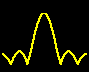6. Frequency Response of FIR Filters4

The goal of this lab is to study the frequency response. For FIR filters this is the response to inputs such as complex exponentials and sinusoids. You can use firfilt(), or conv(), to implement filters and freqz() to obtain the filter’s frequency response. As a result, you should learn how to characterize a filter by knowing how it reacts to different frequency components in the input.
The goal of this lab is to study the response of FIR filters to inputs such as complex exponentials and sinusoids. In the experiments of this lab, you will use firfilt(), or conv(), to implement filters and freqz() to obtain the filter's frequency response. As a result, you should learn how to characterize a filter by knowing how it reacts to different frequency components in the input. This lab also introduces two practical filters: bandpass filters and nulling filters. Bandpass filters can be used to detect and extract information from sinusoidal signals, e.g., tones in a touch-tone telephone dialer. Nulling filters can be used to remove sinusoidal interference, e.g., jamming signals in a radar.
The goal of this lab is to study the sinusoidal response of some simple FIR filters in Matlab. This leads to a study of the frequency response function. In the experiments of this lab, you will use the Matlab GUI called dltidemo to find the frequency response function for FIR filters. [Files]
In this mini-project you will write a simple Matlab program that removes unwanted tones from a wav file. The file SunshineSquare.wav has had some unwanted tones added to it. Your job is to remove the tones so you can hear the message better. [Files]

####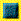7. Discrete-Time Fourier Transform2

This lab introduces a practical application where sinusoidal signals are used to transmit information: a touch-tone dialer. Bandpass FIR filters can be used to extract the information encoded in the waveforms. The goal of this lab is to design and implement bandpass FIR filters in M ATLAB , and to do the decoding automatically. In the experiments of this lab, you will use firfilt(), or conv(), to implement filters and freqz() to obtain the filter’s frequency response. 1 As a result, you should learn how to characterize a filter by knowing how it reacts to different frequency components in the input. [Files]
The goal of this lab is to learn some methods for designing practical FIR filters in Matlab. These filters will have a finite number of coefficients, and a frequency response that approximates an ideal frequency response shape.

####8. Discrete Fourier Transform2

The objective of this lab is to introduce more complicated signals that are related to the basic sinusoid. These signals which implement frequency modulation (FM) and amplitude modulation (AM) are widely used in communication systems such as radio and television. In addition, they can be used to create interesting sounds that mimic musical instruments. The resulting signal can be analyzed to show its time-frequency behavior by using the spectrogram. This lab studies signal synthesis for AM and FM signals, and their time-frequency content as shown in a spectrogram. An underlying objective of the lab is to learn more about the spectrogram.
The objective of this lab is to study further the spectral content of signals analyzed via the spectrogram. There are several specific steps that will be considered in this lab:
1. Synthesize a linear-FM chirp with a Matlab M-file, and display its spectrogram. Choose the chirp parameters so that aliasing will happen.
2. Synthesize a periodic triangle wave with a Matlab M-file, and display its spectrogram. Relate the harmonic line spectrum to the fundamental period of the triangle wave.
3. Compare spectrograms using different scales for amplitude: decibels (dB) for amplitude versus linear amplitude.
4. Examine details of the harmonic lines in the dB spectrogram of the triangle wave.
5. Spectrogram: make a spectrogram of your voice signal, and relate the harmonic line spectrum to your previous measurement of pitch period.

####9. z-Transforms2

This lab introduces a practical application where sinusoidal signals are used to transmit information: a touch-tone dialer. Bandpass FIR filters can be used to extract the information encoded in the waveforms. The goal of this lab is to design and implement bandpass FIR filters in Matlab, and to do the decoding automatically. In the experiments of this lab, you will use firfilt(), or conv(), to implement filters and freqz() to obtain the filter’s frequency response. As a result, you should learn how to characterize a filter by knowing how it reacts to different frequency components in the input. [Files]
This lab introduces a practical application where we attempt to extract information from sinusoidal signals - in this case, piano notes. Bandpass FIR filters can be used to extract the information encoded in the waveforms. The goal of this lab is to design and implement several bandpass FIR filters in Matlab, and use the filtered outputs to determine automatically which note is being played. However, since there are 88 keys on the piano, we will only require the system to figure out which octave the note is in, not the exact note. In the experiments of this lab, you will use firfilt(), or conv(), to implement filters and freqz() to obtain the filter's frequency response. As a result, you should learn how to characterize a filter by knowing how it reacts to different frequency components in the input. [Files]

####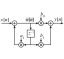10. IIR Filters6

The objective for this lab is to build an intuitive understanding of the relationship between the location of poles and zeros in the z-domain, the impulse response $$h[n]$$ in the $$n$$-domain, and the frequency response $$H(e^{j\hat\omega})$$ (the $$\hat\omega$$-domain). A graphical user interface (GUI) called PeZ was written in MATLAB for doing interactive explorations of the three domains.
The goal of this lab is to explore the connection between the time domain $$(n)$$, the frequency domain ($$\hat\omega$$), and the $$z$$-transform domain, using the GUI PeZ in Matlab.
1. Placing Zeros: When placed on the unit circle, zeros of the numerator $$B(z)$$ will force the frequency response to be zero which can then be used to null out sinusoids at one frequency.
2. Placing Poles: When placed near the unit circle (but inside), roots of denominator $$A(z)$$ will create peaks in the frequency response which can be used to form BPFs.
3. Designing IIR Notch Filters: requires conjugate zeros on the unit circle (UC) with a poles at the same angle, just inside the UC. The frequency response of the notch is much sharper than a nulling filter which is an FIR filter formed only from the conjugate zeros on the UC.
In this mini-project you will experiment with PeZ to learn the connection between pole-zero placement and frequency response. Given this information you will redo the Tone Removal Mini-Project using an IIR.
For this mini project you will write a simple function that listens to a tone and identifies what note it is. [Files]
For this mini project you will write a simple function that listens to a wav file of a simple song and identifies the notes being played. The wav files have some simple songs on which you can practice. [Files]
You have gotten to the point in your studies that you can understand DSP papers that appear in IEEE publications. The purpose of this project is to read one such paper and reproduce some of its results.